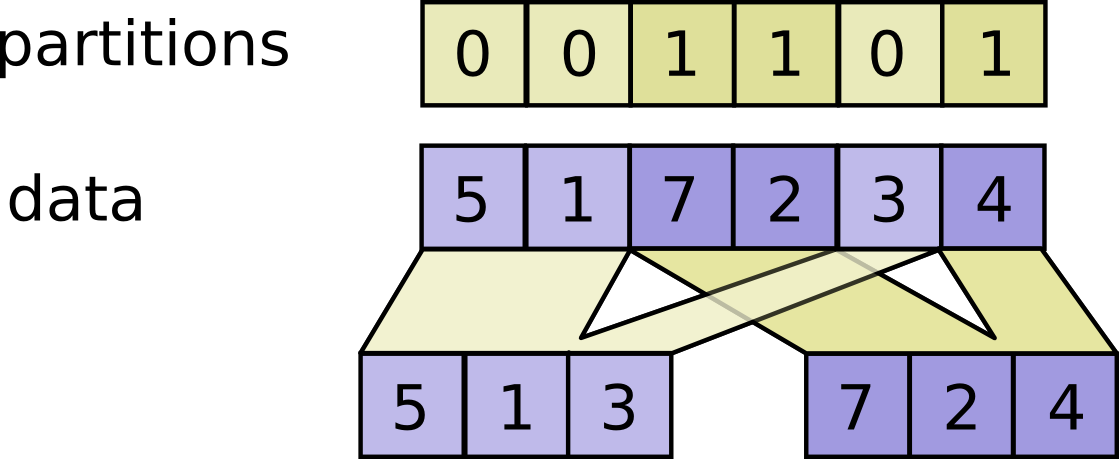Help protect the Great Barrier Reef with TensorFlow on Kaggle

tf.dynamic_partition

Partitions data into num_partitions tensors using indices from partitions.

For each index tuple js of size partitions.ndim, the slice data[js, ...] becomes part of outputs[partitions[js]]. The slices with partitions[js] = i are placed in outputs[i] in lexicographic order of js, and the first dimension of outputs[i] is the number of entries in partitions equal to i. In detail,

outputs[i].shape = [sum(partitions == i)] + data.shape[partitions.ndim:]

outputs[i] = pack([data[js, ...] for js if partitions[js] == i])

For example:

# Scalar partitions.
partitions = 1
num_partitions = 2
data = [10, 20]
outputs = []  # Empty with shape [0, 2]
outputs = [[10, 20]]

# Vector partitions.
partitions = [0, 0, 1, 1, 0]
num_partitions = 2
data = [10, 20, 30, 40, 50]
outputs = [10, 20, 50]
outputs = [30, 40]

See dynamic_stitch for an example on how to merge partitions back.data A Tensor.
partitions A Tensor of type int32. Any shape. Indices in the range [0, num_partitions).
num_partitions An int that is >= 1. The number of partitions to output.
name A name for the operation (optional).

A list of num_partitions Tensor objects with the same type as data.

[{ "type": "thumb-down", "id": "missingTheInformationINeed", "label":"Missing the information I need" },{ "type": "thumb-down", "id": "tooComplicatedTooManySteps", "label":"Too complicated / too many steps" },{ "type": "thumb-down", "id": "outOfDate", "label":"Out of date" },{ "type": "thumb-down", "id": "samplesCodeIssue", "label":"Samples / code issue" },{ "type": "thumb-down", "id": "otherDown", "label":"Other" }]
[{ "type": "thumb-up", "id": "easyToUnderstand", "label":"Easy to understand" },{ "type": "thumb-up", "id": "solvedMyProblem", "label":"Solved my problem" },{ "type": "thumb-up", "id": "otherUp", "label":"Other" }]